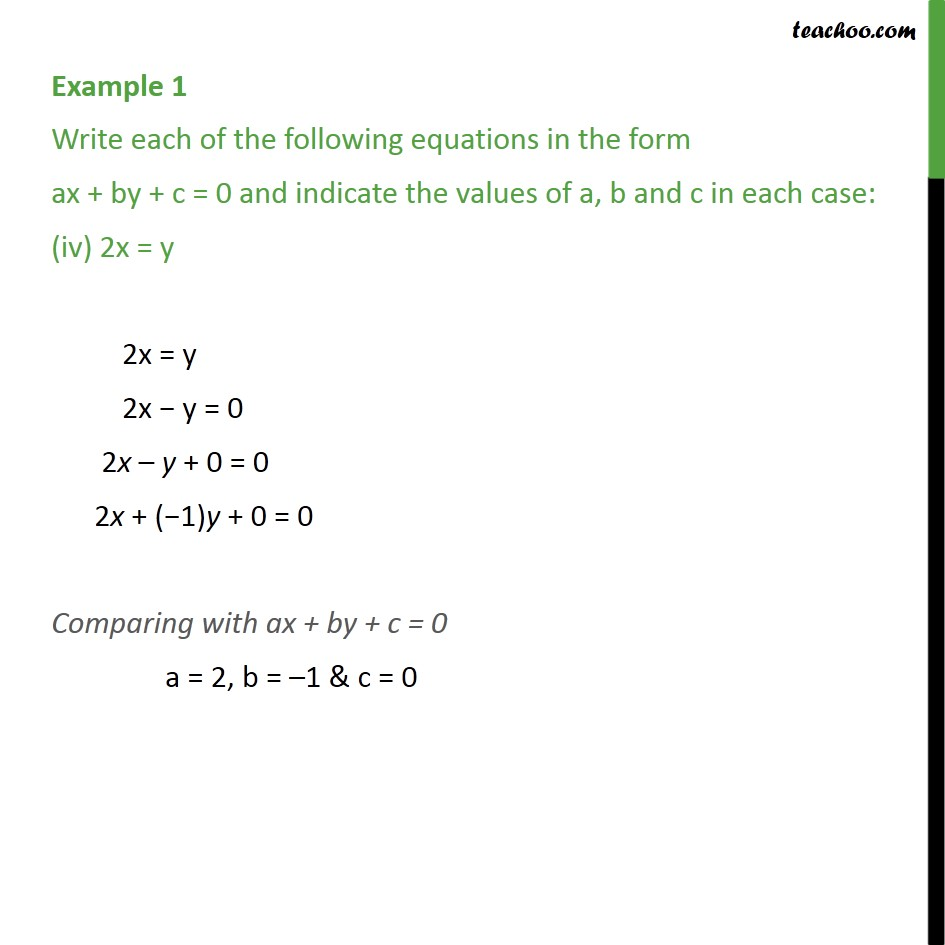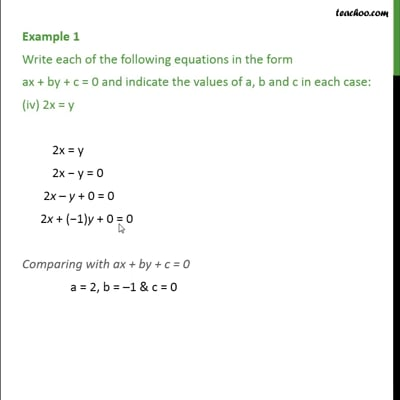Examples

Chapter 4 Class 9 Linear Equations in Two Variables
Serial order wiseThis video is only available for Teachoo black users

Maths Crash Course - Live lectures + all videos + Real time Doubt solving!

### Transcript

Example 1 Write each of the following equations in the form ax + by + c = 0 and indicate the values of a, b and c in each case: (iv) 2x = y 2x = y 2x − y = 0 2x – y + 0 = 0 2x + (−1)y + 0 = 0 Comparing with ax + by + c = 0 a = 2, b = –1 & c = 0